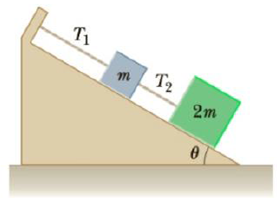Chapter 4, Problem 57P

Chapter
Section
Textbook Problem

# Two blocks of masses m and 2m are held in equilibrium on a frictionless incline as in Figure P4.57. In terms of m and θ, find (a) the magnitude of the tension T1 in the upper cord and (b) the magnitude of the tension T2 in the lower cord connecting the two blocks.Figure P4.57

(a)

To determine
The tension on the upper cord.

Explanation

Given Info: Mass of the upper block is m. Mass of the lower block is 2m.

The free body diagram is given below.

• N1 and N2 are the normal forces.
• m and 2m are masses of the blocks.
• T1 and T2 are the tension on the upper and the lower cords.
• g is the acceleration due to gravity.
• θ is the angle of inclination

(b)

To determine
The tension on the lower cord.

### Still sussing out bartleby?

Check out a sample textbook solution.

See a sample solution

#### The Solution to Your Study Problems

Bartleby provides explanations to thousands of textbook problems written by our experts, many with advanced degrees!

Get Started

## Additional Science Solutions

#### Find more solutions based on key concepts